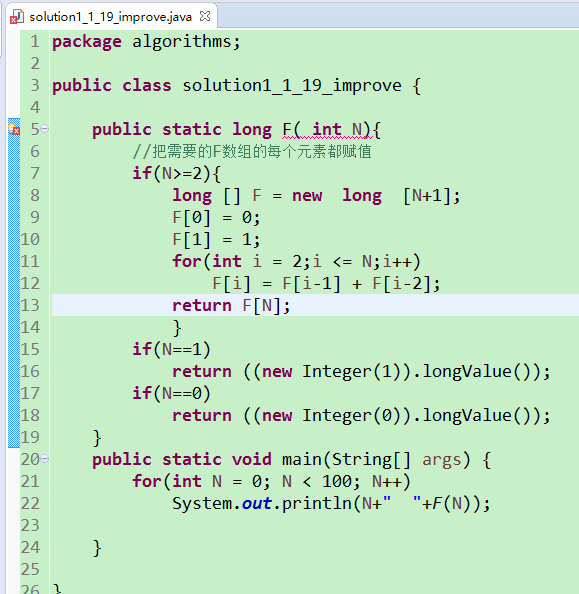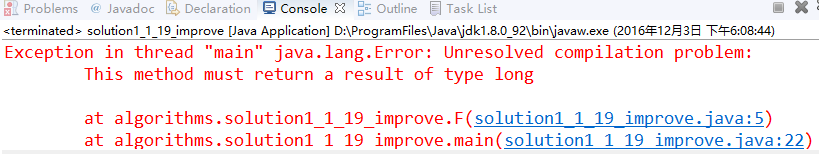Java 方法返回值类型 类型转换renturn 1 ; 这个 1 会被自动转化为long类型吗

return （long ）1;

9个回答

****问题1**.** 方法F（）缺少返回值，如果if都不满足时的返回值，应该在方法F（） 最后一行补上一个return 1或return 100或return N。

public class test{
public static long F(long N){
if(N>=2){
long[] F=new long [(int) N+1];
F=0;
F=1;
for(int i=2;i<=N;i++)

``````            F[i]=F[i-1]+F[i-2];
return F[(int)N];
}
if(N==1) return ((new Integer(1).longValue()));
if(N==0) return ((new Integer(0).longValue()));
return 100;
}

public static void main(String[] args) {
// TODO Auto-generated method stub
for(long N=0;N<10;N++)
System.out.println(N+","+F(N));
}
``````

}